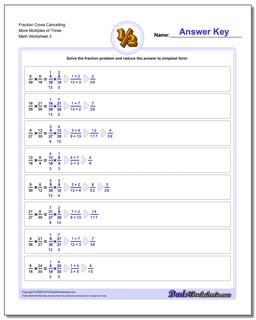# Math Worksheets: Fraction Multiplication: Fraction Multiplication: Fraction Cross Cancelling More Multiples of Three (Third Worksheet)## Fraction Cross Cancelling More Multiples of Three (Third Worksheet)

PropertyValue
DescriptionFraction Cross Cancelling More Multiples of Three: The multiplying fraction worksheets in this section have a heavy emphasis on problems that require you to cross multiply (or cross cancel) to get the product. (Third Worksheet)
Resource TypeWorksheet